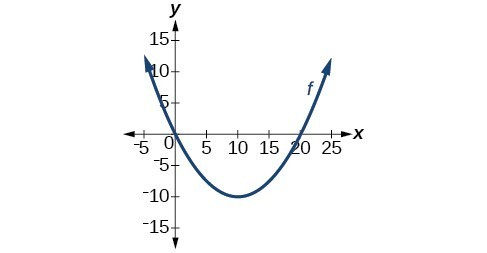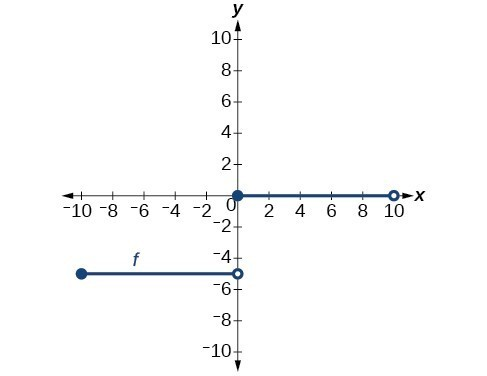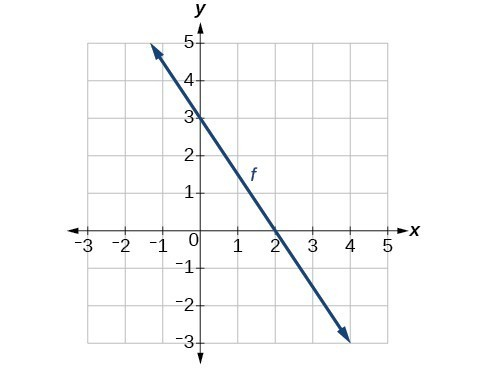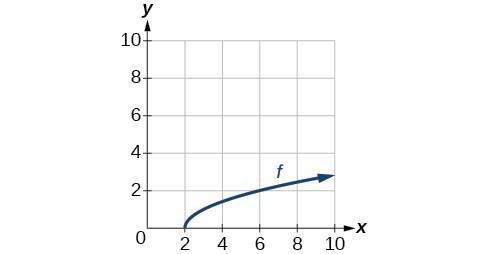## Section Exercises

1. Describe why the horizontal line test is an effective way to determine whether a function is one-to-one?

2. Why do we restrict the domain of the function $f\left(x\right)={x}^{2}$ to find the function’s inverse?

3. Can a function be its own inverse? Explain.

4. Are one-to-one functions either always increasing or always decreasing? Why or why not?

5. How do you find the inverse of a function algebraically?

6. Show that the function $f\left(x\right)=a-x$ is its own inverse for all real numbers $a$.

For the following exercises, find ${f}^{-1}\left(x\right)$ for each function.

7. $f\left(x\right)=x+3$

8. $f\left(x\right)=x+5$

9. $f\left(x\right)=2-x$

10. $f\left(x\right)=3-x$

11. $f\left(x\right)=\frac{x}{x+2}\\$

12. $f\left(x\right)=\frac{2x+3}{5x+4}$

For the following exercises, find a domain on which each function $f$ is one-to-one and non-decreasing. Write the domain in interval notation. Then find the inverse of $f$ restricted to that domain.

13. $f\left(x\right)={\left(x+7\right)}^{2}$

14. $f\left(x\right)={\left(x - 6\right)}^{2}$

15. $f\left(x\right)={x}^{2}-5$

16. Given $f\left(x\right)=\frac{x}{2}+x\\$ and $g\left(x\right)=\frac{2x}{1-x}\\$

a. Find $f\left(g\left(x\right)\right)$ and $g\left(f\left(x\right)\right)\\$

b. What does the answer tell us about the relationship between $f\left(x\right)$ and $g\left(x\right)?$

For the following exercises, use function composition to verify that $f\left(x\right)$ and $g\left(x\right)$ are inverse functions.

17. $f\left(x\right)=\sqrt{x - 1}$ and $g\left(x\right)={x}^{3}+1$

18. $f\left(x\right)=-3x+5$ and $g\left(x\right)=\frac{x - 5}{-3}$

For the following exercises, use a graphing utility to determine whether each function is one-to-one.

19. $f\left(x\right)=\sqrt{x}$

20. $f\left(x\right)=\sqrt{3x+1}$

21. $f\left(x\right)=-5x+1$

22. $f\left(x\right)={x}^{3}-27$

For the following exercises, determine whether the graph represents a one-to-one function.

23.24.For the following exercises, use the graph of $f$ shown in [link].25. Find $f\left(0\right)$.

26. Solve $f\left(x\right)=0$.

27. Find ${f}^{-1}\left(0\right)$.

28. Solve ${f}^{-1}\left(x\right)=0$.

For the following exercises, use the graph of the one-to-one function shown below.29. Sketch the graph of ${f}^{-1}$.

30. Find $f\left(6\right)\text{ and }{f}^{-1}\left(2\right)$.

31. If the complete graph of $f$ is shown, find the domain of $f$.

32. If the complete graph of $f$ is shown, find the range of $f$.

For the following exercises, evaluate or solve, assuming that the function $f$ is one-to-one.

33. If $f\left(6\right)=7$, find ${f}^{-1}\left(7\right)$.

34. If $f\left(3\right)=2$, find ${f}^{-1}\left(2\right)$.

35. If ${f}^{-1}\left(-4\right)=-8$, find $f\left(-8\right)$.

36. If ${f}^{-1}\left(-2\right)=-1$, find $f\left(-1\right)$.

For the following exercises, use the values listed in the table below to evaluate or solve.

 $x$ $f\left(x\right)$ 0 8 1 0 2 7 3 4 4 2 5 6 6 5 7 3 8 9 9 1

37. Find $f\left(1\right)$.

38. Solve $f\left(x\right)=3$.

39. Find ${f}^{-1}\left(0\right)$.

40. Solve ${f}^{-1}\left(x\right)=7$.

41. Use the tabular representation of $f$ to create a table for ${f}^{-1}\left(x\right)$.

 $x$ 3 6 9 13 14 $f\left(x\right)$ 1 4 7 12 16

For the following exercises, find the inverse function. Then, graph the function and its inverse.

42. $f\left(x\right)=\frac{3}{x - 2}$

43. $f\left(x\right)={x}^{3}-1$

44. Find the inverse function of $f\left(x\right)=\frac{1}{x - 1}$. Use a graphing utility to find its domain and range. Write the domain and range in interval notation.

45. To convert from $x$ degrees Celsius to $y$ degrees Fahrenheit, we use the formula $f\left(x\right)=\frac{9}{5}x+32$. Find the inverse function, if it exists, and explain its meaning.

46. The circumference $C$ of a circle is a function of its radius given by $C\left(r\right)=2\pi r$. Express the radius of a circle as a function of its circumference. Call this function $r\left(C\right)$. Find $r\left(36\pi \right)$ and interpret its meaning.

47. A car travels at a constant speed of 50 miles per hour. The distance the car travels in miles is a function of time, $t$, in hours given by $d\left(t\right)=50t$. Find the inverse function by expressing the time of travel in terms of the distance traveled. Call this function $t\left(d\right)$. Find $t\left(180\right)$ and interpret its meaning.